SSC

## SSC Practical 2023

A notice has been issued by the Department of Secondary and Higher Secondary Education regarding the submission of the practical book of the candidate participating in the SSC examination of 2023. The notice mentions the practical application book syllabus of SSC candidates for 2023and what to do in practice. Although the Sanskrit syllabus is the SSC examination of 2023, the students have to submit their elective subjects in the practical register of the grain institution. SSC Practical 2023## SSC 2023 Practical Question

However, there is no need to submit the practical book of the fourth subject. In case of submission of a practical book, any two of the chapters mentioned in the short syllabus should be submitted to the respective institutions. If a student has done practicals before and if his book contains two practicals of a short syllabus, then he can submit that practical book.

## SSC Practical Syllabus 2023

The Department of Education has asked the SSC/equivalent candidates for the 2023 SSC/equivalent examination to submit only the practical subject book of the elective subjects in which the examination is to be held.

## SSC Practical Khata PDF 2023

Samples of all the practical books of the candidates appearing for the SSC examination in 2023 will be available from our website. Following the samples and completing the practical book will not be a problem in practice.Students of SSC 2023 will have to do any two practicals as per their short syllabus. After completing the test work in the laboratory, its details, observations, and results should be written in the practical notebook. Draw a parallel straight line for writing on the right page of the notebook and leave blank for drawing the design of equipment etc. on the page.

SSC Short Syllabus 2023 PDF Download । এস এস সি সংক্ষিপ্ত সিলেবাস ২০২১। eduinfobd.com

Students need to follow the instructions below to write a practical notebook

1. On the right side of the margin and at the top in capital letters is the subject of the test work and in the upper left corner of the margin to the left of the test performance data.
2. The past participle must be used when writing a notebook
3. The description should be short but care should be taken not to omit any necessary information
4. Write down any prepared method briefly. He will then write the three main titles according to the observation and decision based on the Rafkhata written in his religion laboratory. Exclude irrelevant information and add the only necessary information.
5. Every new test task needs to start writing on a new page.
6. The text on the right page should always be written in ink and the drawing on page no. Should use a wooden pencil.
7. Care should be taken in writing the notebook. The notebook research project should be written neatly and submitted to the respective institutions

## SSC Physics Practical  Answer 2023

In the case of every practical in physics, it is important to determine the accuracy of the measurements and to make sure that the calculations are correct. The practicalities of measurement must be taken seriously and through accurate calculations. Images should be drawn on the left side of the page. On the right side you have to write all the accounts and other information. Care must be taken to ensure that the calculation of the table is correct.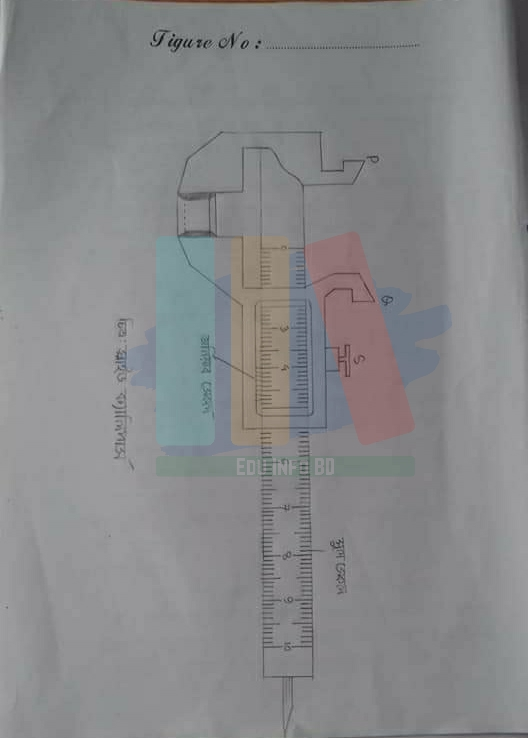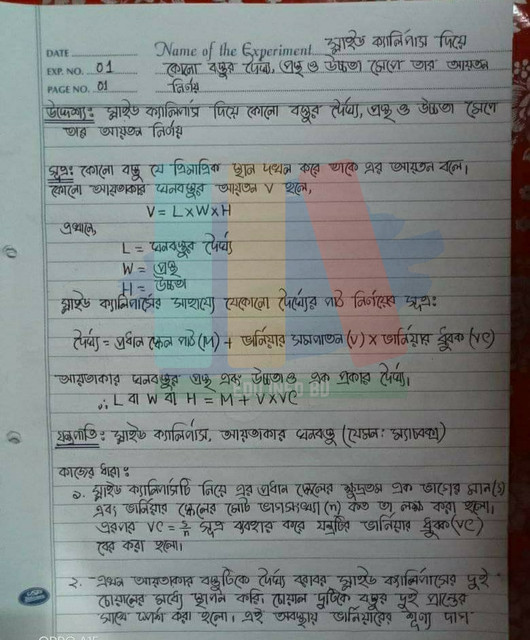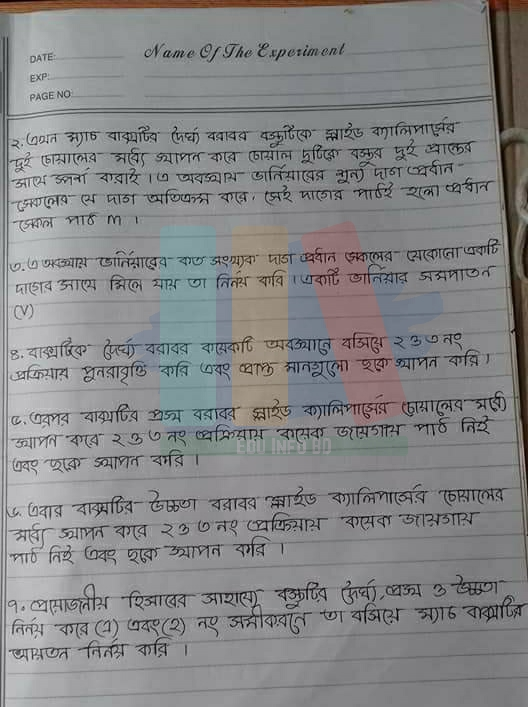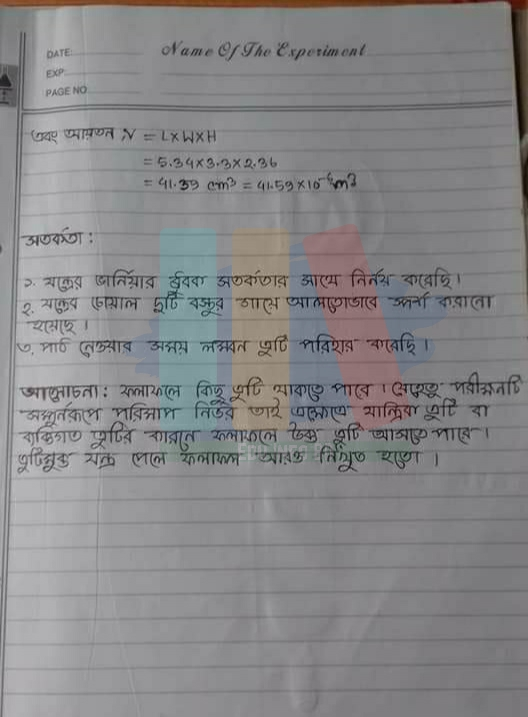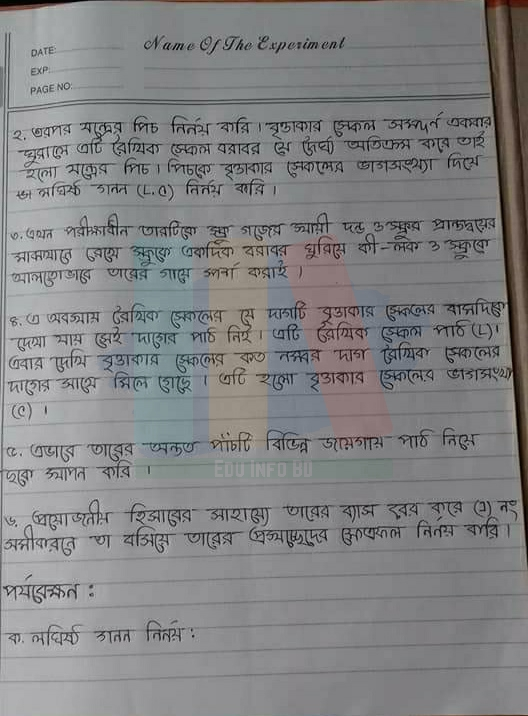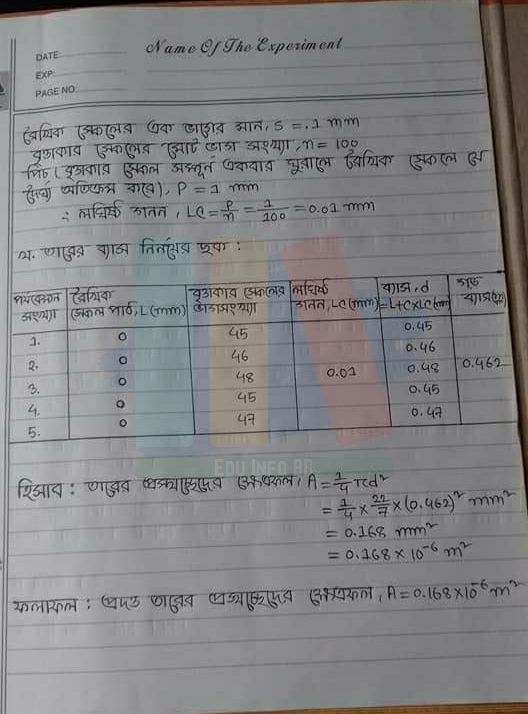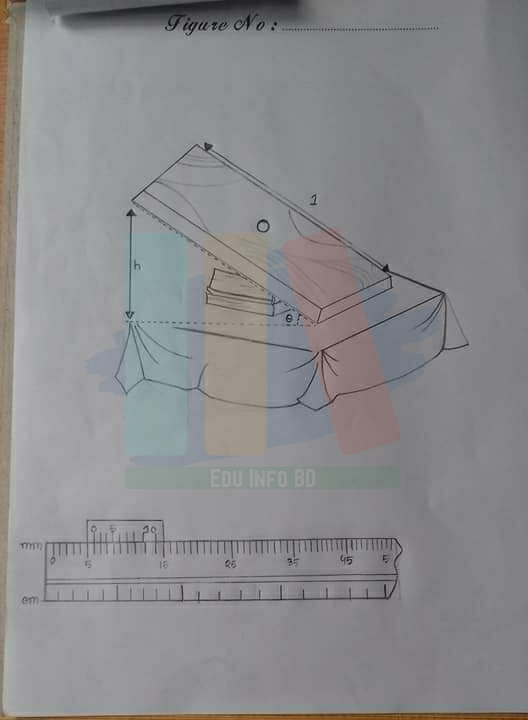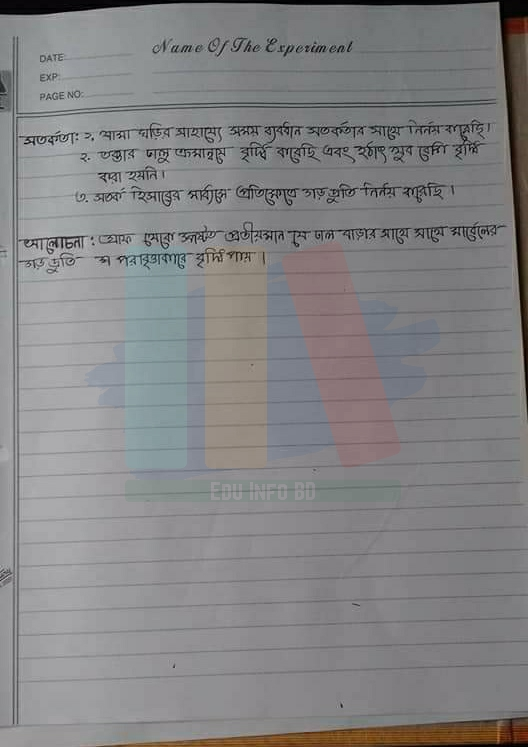## SSC Chemistry Practical  Answer 2023

When writing Chemical Practical, you must write the chemical equations of the element correctly. After writing the equations, you have to describe it in detail. After writing the solutions of chemical equations, one has to check whether they are correct. The pictures should be drawn on the left side and all the calculations of equations etc. should be written on the right side. The writing should be clear and the practical should be done carefully.## SSC Higher Math Practical  Answer 2023

While practicing higher mathematics, many times the solution of mathematics is correct, but the practicality is not correct because the rules are not followed properly. If higher mathematics is one of your main subjects then you have to do the practicals of higher mathematics. In this case, the mathematical solutions need to be verified correctly and re-examined to see if they are correct. This can be done through precision verification. If you have a higher math practical, you have to bring a page of the graph from the back to the front and put it on the left side. The graph should be placed on the left side so that his description can be written as it looks bad while writing. Graphs should always be done with a pencil and written with a pen. The symbols must be mentioned correctly in the case of images.

## SSC Biology Practical  Answer 2023

Figure is a very big factor in Biology Practical. The image must be placed correctly and the image parts must be identified correctly. Make sure that the parts that are to be identified in the figure are clear. The image should be drawn in pencil and it should be drawn on the page designated for the image. On the left is a thick sheet of paper set for the image in the case of Biological Practical. After the image is properly sized, it is necessary to see if it is correct to write its description and in the case of biology, class, tribe, class, etc. When writing a scientific name, you have to mark it down or write it in curved letters. Images should be drawn with a pencil and Biology Practical should be written with a pencil or pen.## How to Write Practical Notebook for SSC 2023 Exam

Rules for writing practical books

There are a few things to keep in mind while writing a practical book and the practical book should be mentioned

• Title
• Introduction
• Materials and methods
• The result
• Discussion
• Conclusion
• References
• Appendix

Rules for writing titles

The subject of the practical report mentioned in the question should be written as the title of the practical. When writing a title, write a clear and small title.

Rules for Introduction Writing

All the information about what is to be written practically, a lot of information has to be written correctly. Introduction It is better to write approximately 200 words.

Materials and methods

Describe what was done in the practical test. The materials used during the application and the follow-up method should also be included. Materials and methods It is best to write approximately 300 words.

The result

The result that can be obtained by practical application, after analyzing all the information, the result can be obtained by calculation is the presentation of the result. The result requires writing 300 words.

Discussion

Discussion is the interpretation of the application and the explanation of the search and their presentation in the context of background information. The discussion part of the practice should be written in at least 500 words.

Conclusion

The results and explanations of the practice are summarized as follows. Write a 200-word conclusion.

References

References are the sources of information used in the report. The reference should be written after the conclusion at the bottom of the practical.

Appendix

Not all types of practice tests are required, but all practical appendices require detailed practical information.

You can also get a complete guideline for writing a practical book from our post below.

The practical book should be completed by correctly mentioning all the points including the above instructions and the title. Figures and tables must be clear. The topics that are given are the content to be presented in practice.